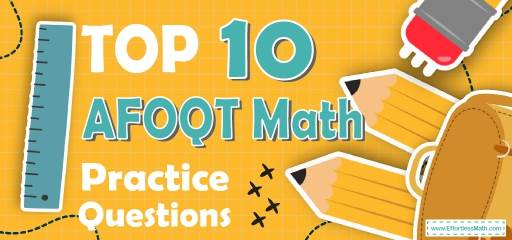# Top 10 AFOQT Math Practice QuestionsPreparing for the AFOQT Math test? Need some sample practice questions to measure your exam readiness? The best way to prepare for your AFOQT Math test is to work through as many AFOQT Math practice questions as possible. Here are the top 10 AFOQT Math practice questions to help you review the most important AFOQT Math concepts. These AFOQT Math practice questions are designed to cover mathematics concepts and topics that are found on the actual test. The questions have been fully updated to reflect the latest 2022 AFOQT guidelines. Answers and full explanations are provided at the end of the post.

Start your AFOQT Math test prep journey right now with these sample AFOQT Math questions.

## AFOQT Math Practice Questions

1- Aria was hired to teach three identical math courses, which entailed being present in the classroom for 36 hours altogether. At $25 per class hour, how much did Aria earn for teaching one course? A.$50

B. $300 C.$600

D. $1,400 2- What is the value of the expression $$(5(x-2y)+(2-x))^2$$ when $$x=3$$ and $$y=-2$$? A. 20 B. 50 C. 1156 D. 36 3- Karen is 9 years older than her sister Michelle, and Michelle is 4 years younger than her brother David. If the sum of their ages is 82, how old is Michelle? A. 21 B. 25 C. 29 D. 23 4- What is the slope of a line that is perpendicular to the line $$4x-2y=12$$? A. $$-2$$ B. 4 C. 2 D. $$-\frac{1}{2}$$ 5- Julie gives 8 pieces of candy to each of her friends. If Julie gives all her candy away, which amount of candy could have been the amount she distributed? A. 187 B. 216 C. 343 D. 223 6- Which of the following is equal to the expression below? $$(2x+2y)(2x-y)$$ A. $$4x^2- 2y^2$$ B. $$2x^2+6xy-2y^2$$ C. $$24x^2+2xy-2y^2$$ D. $$4x^2+2xy-2y^2$$ 7- Each year, a cyber café charges its customers a base rate of$15, with an additional $0.20 per visit for the first 40 visits, and$0.10 for every visit after that. How much does the cyber café charge a customer for a year in which 60 visits are made?

A. $25 B.$29

C. $35 D.$39

8- What is the product of all possible values of $$x$$ in the following equation?
$$|x-10| = 3$$

A. 3

B. 7

C. 13

D. 91

9- What is the value of $$x$$ in the following system of equations?
$$2x+5y=11$$
$$4x-2y=-14$$

A. $$-1$$

B. 1

C. 4

D. $$-2$$

10- While at work, Emma checks her email once every 90 minutes. In 9−hour, how many times does she check her email?

A. 4 Times

B. 5 Times

C. 7 Times

D. 6 Times

## Best AFOQT Math Prep Resource for 2022

1- B
$$36 ÷ 3 = 12$$ hours for one course
$$12 × 25 = 300 ⇒ 300$$

2- D
Plug in the value of $$x$$ and $$y$$.
$$x=3$$ and $$y=-2$$
$$(5(x-2y)+(2-x))^2=(5(3-2(-2))+(2-3))^2=(5(3+4)+(-1))^2 = (34)^2=36$$

3- D
Michelle $$=$$ Karen $$– 9$$
Michelle $$=$$ David $$– 4$$
Karen $$+$$ Michelle $$+$$ David $$= 82$$
Karen $$+ 9 =$$ Michelle ⇒ Karen $$=$$ Michelle $$– 9$$
Karen $$+$$ Michelle $$+$$ David $$= 82$$
Now, replace the ages of Karen and David by Michelle. Then:
Michelle $$+ 9 +$$ Michelle + Michelle $$+ 4 = 82$$
$$3$$Michelle $$+ 13 = 82$$ ⇒ 3Michelle $$= 82 – 13$$
$$3$$Michelle $$= 69$$
Michelle $$= 23$$

4- D
The equation of a line in slope intercept form is: $$y=mx+b$$
Solve for $$y$$.
$$4x-2y=12 {\Rightarrow} -2y=12-4x {\Rightarrow} y=(12-4x){\div}(-2) {\Rightarrow} y=2x-6$$
The slope of this line is $$2$$.
The product of the slopes of two perpendicular lines is $$-1$$.
Therefore, the slope of a line that is perpendicular to this line is:
$$m_{1} {\times} m_{2} = -1 {\Rightarrow} 2 {\times} m_{2} = -1 {\Rightarrow} m_{2} = \frac{-1}{2}$$

5- B
Since Julie gives $$8$$ pieces of candy to each of her friends, then, the number of pieces of candies must be divisible by 8.
A. $$187 ÷ 8 = 23.375$$
B. $$216 ÷ 8 = 27$$
C. $$343 ÷ 8 = 42.875$$
D. $$223 ÷ 8 = 27.875$$
Only choice B gives a whole number.

6- D
Use FOIL method.
$$(2x+2y)(2x-y) = 4x^2-2xy+4xy-2y^2=4x^2+2xy-2y^2$$

7- A
The base rate is $$15$$.
The fee for the first $$40$$ visits is: $$40×0.20=8$$
The fee for the visits $$41$$ to $$60$$ is: $$20×0.10=2$$
Total charge: $$15 + 8 + 2 = 25$$

8- D
To solve absolute values equations, write two equations.
$$x-10$$ could be positive $$3$$, or negative $$3$$. Therefore,
$$x-10=3 \Rightarrow x=13$$
$$x-10=-3 \Rightarrow x=7$$
Find the product of solutions: $$7 \times 13 = 91$$

9- D
Solving Systems of Equations by Elimination
Multiply the first equation by $$(-2)$$, then add it to the second equation.
$${-2(2x+5y= 11) \ 4x-2y=-14} {\Rightarrow} {-4x-10y= -22 \ 4x-2y=-14} {\Rightarrow} {-12y= -36} {\Rightarrow} {y= 3}$$
Plug in the value of y into one of the equations and solve for $$x$$.
$$2x+5(3)= 11 {\Rightarrow} 2x+15= 11 {\Rightarrow} 2x= -4 {\Rightarrow} x= -2$$

10- D
Change $$9$$ hours to minutes, then: $$9 × 60 = 540$$ minutes
$$\frac{540}{90}=6$$

Looking for the best resource to help you succeed on the AFOQT Math test?

## More from Effortless Math for AFOQT Test …

### How do I prepare for the AFOQT Math?

Get your answer in this post: How to Prepare for the AFOQT Math Test?

### Need FREE online resources for the AFOQT Math test?

Here is our complete list of Top 10 Websites for FREE AFOQT Math Preparation.

Check out our Full-Length AFOQT Math Practice Test and Free AFOQT Math Practice Test.

## Have any questions about the AFOQT Test?

### What people say about "Top 10 AFOQT Math Practice Questions - Effortless Math: We Help Students Learn to LOVE Mathematics"?

No one replied yet.

X
49% OFF

Limited time only!

Save Over 49%

SAVE $34 It was$69.99 now it is \$35.99